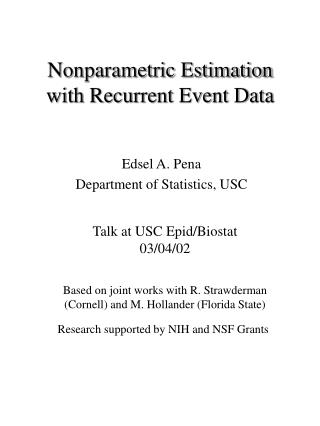DownloadDownload PresentationNonparametric Estimation with Recurrent Event Data

# Nonparametric Estimation with Recurrent Event Data

Télécharger la présentation## Nonparametric Estimation with Recurrent Event Data

- - - - - - - - - - - - - - - - - - - - - - - - - - - E N D - - - - - - - - - - - - - - - - - - - - - - - - - - -
##### Presentation Transcript

1. Nonparametric Estimation with Recurrent Event Data Talk at USC Epid/Biostat 03/04/02 Edsel A. Pena Department of Statistics, USC Based on joint works with R. Strawderman (Cornell) and M. Hollander (Florida State) Research supported by NIH and NSF Grants

2. A Real Recurrent Event Data(Source: Aalen and Husebye (‘91), Statistics in Medicine) Variable:Migrating motor complex (MMC) periods, in minutes, for 19 individuals in a gastroenterology study concerning small bowel motility during fasting state.

3. t-S3=4 T2=59 T1=284 T3=186 S2=343 t=533 S3=529 S1=284 Calendar Scale Pictorial Representation of Data for a Subject • Consider subject #3. • K = 3 • Inter-Event Times, Tj: 284, 59, 186 • Censored Time, t-SK: 4 • Calendar Times, Sj: 284, 343, 529 • Limit of Observation Period: t = 533 0

4. Features of Data Set • Random observation period per subject (administrative, time, resource constraints). • Length of period: t • Event of interest is recurrent. • # of events (K) informative about MMC period distribution (F). • Last MMC period right-censored by which is informative about F. • Calendar times: S1, S2, …, SK.

5. Assumptions and Problem • Aalen and Husebye: “Consecutive MMC periods for each individual appear (to be) approximate renewal processes.” • Translation: The inter-event times Tij’s are assumed stochastically independent. • Problem: Under this IID or renewal assumption, and taking into account the informativeness of K and the right-censoring mechanism, how to estimate the inter-event distribution, F and its parameters (e.g., median).

6. General Form of Data Accrual Calendar Times of Event Occurrences Si0=0 and Sij = Ti1 + Ti2 + … + Tij Number of Events in Observation Period Ki = max{j: Sij<ti} Limits of observation periods, ti’s, may be fixed, or assumed IID with unknown distribution G.

7. Observables Problem Obtain a nonparametric estimator of the inter-event time distribution, F, and determine its properties.

8. Relevance and Applicability • Recurrent phenomena occur in public health/medical settings. • Outbreak of a disease. • Hospitalization of a patient. • Tumor occurrence. • Epileptic seizures. • In other settings. • Non-life insurance claims. • Stock index (e.g., Dow Jones) decreases by at least 6% in a day. • Labor strikes. • Reliability and engineering.

9. Existing Methods and Their Limitations • Consider only the first, possibly right-censored, observation per unit and use the product-limit estimator (PLE). • Loss of information • Inefficient • Ignore the right-censored last observation, and use empirical distribution function (EDF). • Leads to bias (“biased sampling”) • Estimator inconsistent

10. Review: Complete Data • T1, T2, …, Tn IID F(t) = P(T < t) Empirical Survivor Func. (EDF) Asymptotics of EDF

11. In Hazards View Hazard rate function Cumulative hazard function Product representation

12. Alternative Representations EDF in Product Form Another representation of the variance

13. Review: Right-Censored Data • Failure times: T1, T2, …, Tn IID F • Censoring times: C1, C2, …, Cn IID G • Right-censored data (Z1, d1), (Z2, d2), …, (Zn, dn) Zi = min(Ti, Ci) di = I{Ti< Ci} Product-Limit Estimator

14. PLE Properties • Asymptotics of PLE • If G(w) = 0, so no censoring,

15. Recurrent Event Setting • Ni(s,t) = number of events for the ith unit in calendar period [0,s] with inter-event times at most t. • Yi(s,t) = number of events for the ith unit which are known during the calendar period [0,s] to have inter-event times at least t. • Ki(s) = number of events for the ith unit that occurred in [0,s]

16. For MMC Unit #3 Inter-Event Time t=100 t=50 284 343 529 =533 Calendar Time s=550 s=400 K3(s=400) = 2 N3(s=400,t=100) = 1 Y3(s=400,t=100) = 1 t s K3(s=550) = 3 N3(s=550,t=50) = 0 Y3(s=550,t=50) = 3

17. Aggregated Processes Limit Processes as s Increases

18. Estimator of F in Recurrent Event Setting Estimator is called the GPLE; generalizes the EDF and the PLE discussed earlier.

19. Properties of GPLE

20. Asymptotic Distribution of GPLE For large n and regularity conditions, with variance function

21. EDF: • PLE: • GPLE (recurrent event): Variance Functions: Comparisons • If in stationary state, R(t) = t/mF, so mG(w) = mean residual life of t at w.

22. Wang-Chang Estimator (JASA, ‘99) • Can handle correlated inter-event times. Comparison with GPLE may be unfair to WC estimator under the IID setting!

23. Frailty (“Random Effects”) Model • U1, U2, …, Un are IID unobservedGamma(a, a) random variables, called frailties. • Given Ui = u, (Ti1, Ti2, Ti3, …) are independent inter-event times with • Marginal survivor function of Tij:

24. Estimator under Frailty Model • Frailty parameter, a: dependence parameter. • Small (Large) a: Strong (Weak) dependence. • EM algorithm needed to obtain the estimator (FRMLE). Unobserved frailties viewed as missing.GPLE needed in EM algorithm. • Under frailty model: GPLE not consistent when frailty parameter is finite.

25. Comparisons of Estimators • Under gamma frailty model. • F = EXP(q): q = 6 • G = EXP(h): h = 1 • n = 50 • # of Replications = 1000 • Frailty parameter a took values: { (IID Model), 6, 2} • Computer programs: S-Plus and Fortran routines.

26. Simulated Comparison of the Three Estimators for Varying Frailty Parameter Black=GPLE; Blue=WCPLE; Red=FRMLE IID

27. Effect of the Frailty Parameter (a) for Each of the Three Estimators (Black=Infty; Blue=6; Red=2)

28. The Three Estimates of MMC Period Survivor Function IID assumption seems acceptable. Estimate of frailty parameter a is 10.2.